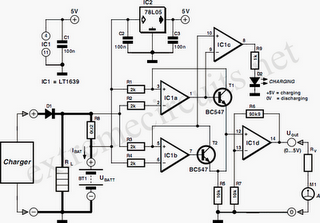# Learning Electronics

Learn to build electronic circuits

# Battery Charger Display Using LT1639

The Over-the-Top type of operational amplifier is ideal for use as a current sense for battery charger applications. The design described here can be used with chargers for rechargeable batteries (Lead/acid or NiCd etc). The 5 V operating supply for the circuit is derived from the battery on charge. The circuit uses a sense resistor R8 to determine the value of current ﬂowing in or out of the battery. An LED output shows whether the battery is charging or discharging and an analogue output displays the battery charge or discharge current. The circuit can also be altered to shown different ranges of charging current to cater for higher capacity cells. IC1a and IC1b together with T1 and T2 form two current sources, which produce a voltage across R5. The voltage across R5 is proportional to the current through resistors R8 and R1 (for IC1a) or R8-R3 (for IC1b).The current source formed by IC1a and T1 is active when the batteries are discharging and IC1b and T2 is active when the batteries are being charged. In each case the inactive opamp will have 0V at its output and the corresponding transistor will be switched off. IC1d amplifies the voltage across R5, which is proportional to the sense current. The component values given in the diagram produce an amplification factor or 10. A sense current of 0.1 A will produce an output voltage of +1 V. The supply voltage to the circuit is +5 V so this will be the maximum value that the output can achieve. This corresponds to a maximum charge/discharge current of 0.5 A To display currents from 0 to 5.0 A, resistor R7 can be omitted to give IC1d a voltage gain of 1.

Higher currents can be displayed by using a lower value of sense resistor R8. A DVM or analogue meter can be used at Vout to give a display of the charge/discharge current. The constant current sources can only function correctly when the supply to the voltage regulator circuit (UBatt. e.g. 6V or 12V) is greater than the operating voltage of the opamps (+5 V). The supply voltage to the LT1639 can be in the range of +3 V and +44V and voltages up to 40V over the supply voltage are acceptable at the inputs to the opamp. IC1c controls the charging/discharging LED output. The inputs to this opamp are connected to the outputs of the current source opamps and its output goes high when the battery is being charged and low when it is discharging.